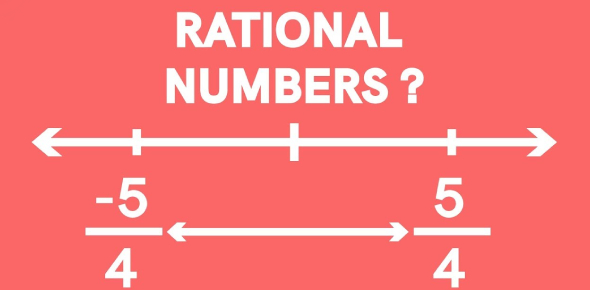# Grade 8: Unit 1 - Rational Numbers

10 Questions | Attempts: 6343
ShareSettingsAre you in grade 8 and struggling with the rational numbers concept? Well, worry no more as the quiz below is set to help you out with this. After this, it will be much easier. All the best.

• 1.
Explain the process for converting a decimal to a fractional equivalent.
• 2.
Explain the process for converting a fraction to a decimal equivalent.
• 3.
Create a real-world rational number problem.  Explain in detail the process of how to solve it and provide both written and numerical explanation.
• 4.
Identify all of the rational numbers from those listed below.
• A.

7

• B.
• C.

3.415

• D.
• E.
• F.
• G.
• 5.
Please answer the following question in the space below. What is the decimal equivalent of ?
• 6.
Please answer the following question in the space below. What is the decimal equivalent of  to the thousandths place?
• 7.
What is the fractional equivalent of 0.333? Note: Enter your fraction as a/b, where a is the numerator and b is the denominator.
• 8.
What is the fractional equivalent of 0.125?Note: enter your fraction as a/b, where a is the numerator and b is the denominator.

## Related TopicsBack to top
×

Wait!
Here's an interesting quiz for you.